# JAC Class 10 Maths Solutions Chapter 6 Triangles Ex 6.3

Jharkhand Board JAC Class 10 Maths Solutions Chapter 6 Triangles Ex 6.3 Textbook Exercise Questions and Answers.

## JAC Board Class 10 Maths Solutions Chapter 6 Triangles Exercise 6.3

Question 1.
State which pairs of triangles in the given figure are similar. Write the similarity criterion used by you for answering the question and also write the pairs of similar triangles in the symbolic form:
1.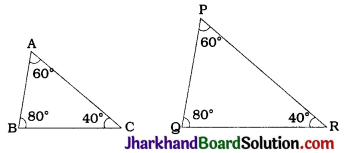In ΔABC and ΔPQR,
∠A = ∠P = 60°, ∠B = ∠Q = 80° and
∠C = ∠R = 40°
∴ By AAA criterion, ΔABC – ΔPQR.

2.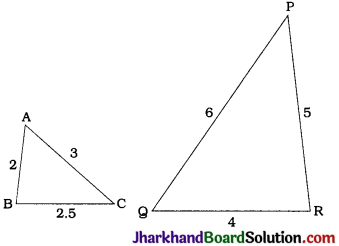In ΔABC and ΔQRP,
$$\frac{\mathrm{AB}}{\mathrm{QR}}=\frac{2}{4}=\frac{1}{2}$$, $$\frac{\mathrm{BC}}{\mathrm{RP}}=\frac{2.5}{5}=\frac{1}{2}$$ and $$\frac{\mathrm{CA}}{\mathrm{PQ}}=\frac{3}{6}=\frac{1}{2}$$
Thus, $$\frac{AB}{QR}=\frac{BC}{RP}=\frac{CA}{PQ}$$
∴ By SSS criterion, ΔABC ~ ΔQRP.

3.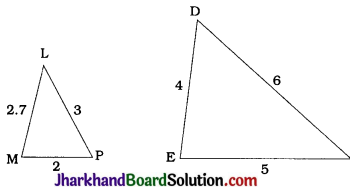No, the given triangles are not similar as
$$\frac{MP}{DE}=\frac{1}{2}$$, $$\frac{LP}{DF}=\frac{1}{2}$$, but $$\frac{LM}{EF}$$ = $$\frac{2.7}{5}≠\frac{1}{2}$$

4.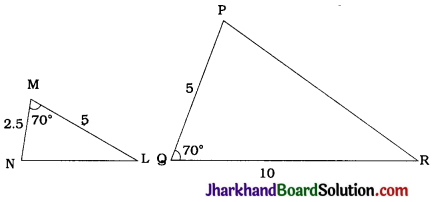In ΔMNL and ΔQPR,
$$\frac{\mathrm{MN}}{\mathrm{QP}}=\frac{2.5}{5}=\frac{1}{2}$$, $$\frac{\mathrm{ML}}{\mathrm{QR}}=\frac{5}{10}=\frac{1}{2}$$
∠M = ∠Q = 70°
∴ By SAS criterion, ΔMNL ~ ΔQPR.

5.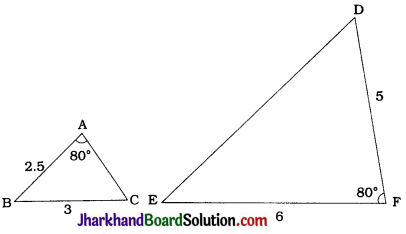No, the given triangles are not similar as two given corresponding sides are proportionate but the corresponding included angles are not equal.

6.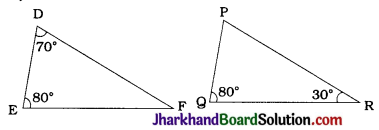In ΔDEF, ∠D = 70°, ∠E = 80°
∠F = 180° – 70° – 80° = 30°
In ΔPQR, ∠Q = 80°, ∠R = 30°
∴ ∠P = 180° – 80° – 30° = 70°
Thus, in ΔDEF and ΔPQR.
∠D = ∠P, ∠E = ∠Q and ∠F = ∠R
∴ By AAA criterion, ΔDEF ~ ΔPQR.Question 2.
In the given figure ΔODC – ΔOBA, ∠BOC = 125° and ∠CDO = 70°. Find ∠DOC, ∠DCO and ∠OAB.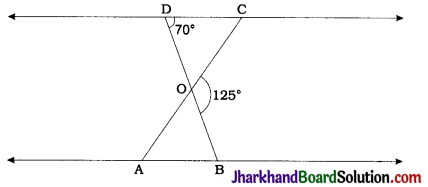In ΔDOC, ∠COB is an exterior angle.
∴ ∠COB + ∠DOC = 180°
∴ 125° + ∠DOC = 180°
∴ ∠DOC = 55°
Again, ∠COB = ∠ODC + ∠DCO
∴ 125° = 70° + ∠DCO
∴ ∠DCO = 55°
Now, ΔODC ~ ΔOBA
∴ ∠OAB = ∠OCD
∴ ∠OAB = 55°
Thus, ∠DOC = 55°, ∠DCO = 55° and
∴ ∠OAB = 55°.

Question 3.
Diagonal AC and BD of a trapezium ABCD with AB || DC intersect each other at the point O. Using a similarity criterion for two triangles, show that $$\frac{OA}{OC}=\frac{OB}{OD}$$
Given:
In trapezium ABCD, AB || DC and diagonals AC and BD intersect at O.
To prove : $$\frac{OA}{OC}=\frac{OB}{OD}$$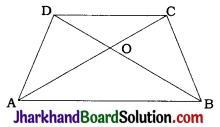Proof: In trapezium ABCD, AB || CD.
∴ ∠CAB = ∠ACD and ∠DBA = ∠BDC
(Alternate angles) ……(1)
Then, in ΔOAB and ΔOCD.
∠OAB = ∠OCD and ∠OBA = ∠ODC [By (1)]
∴ By AA criterion, ΔOAB ~ ΔOCD.
∴ $$\frac{OA}{OC}=\frac{OB}{OD}$$

Question 4.
In the given figure $$\frac{QR}{QS}=\frac{QT}{PR}$$ and ∠1 = ∠2. Show that ΔPQS ~ ΔTQR.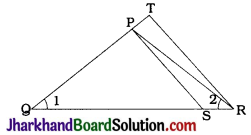In ΔPQR, ∠1 = ∠2, i.e., ∠PQR = ∠PRQ
∴ PR = QP
Now, $$\frac{QR}{QS}=\frac{QT}{PR}$$
∴ $$\frac{QR}{QS}=\frac{QT}{QP}$$
In ΔTQR, P and S are points on QT and QR respectively and $$\frac{QR}{QS}=\frac{QT}{QP}$$
∴ By theorem 6.2, SP || RT.
∴ ∠QPS = ∠QTR and ∠QSP = ∠QRT (Corresponding angles)
Now, in ΔPQS and ΔTQR.
∠QPS = ∠QTR,
∠QSP = ∠QRT and
∠PQS = ∠TQR (Same angle)
∴ By AAA criterion, ΔPQS ~ ΔTQR.Question 5.
S and T are points on sides PR and QR of ΔPQR such that ∠P = ∠RTS. Show that ΔRPQ ~ ΔRTS
Given:
S and T are points on sides PR and QR of ΔPQR such that ∠P = ∠RTS.
To prove ΔRPQ ~ ΔRTS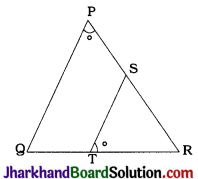Proof : ∠P = ∠RTS
∴∠RPQ = ∠RTS.
In ΔRPQ and ΔRTS,
∠RPQ = ∠RTS and
∠PRQ = ∠TRS (Same angle)
∴ By AA criterion, ΔRPQ ~ ΔRTS.

Question 6.
In the given figure, if ΔABE ≅ ΔACD, show that ΔADE ~ ΔABC.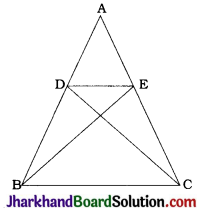ΔABE ≅ ΔACD (Given)
∴ AB = AC and AE = AD (CPCT)
∴ $$\frac{AE}{AC}=\frac{AD}{AB}$$
$$\frac{AE}{AC}=\frac{AD}{AB}$$
and ∠DAE = ∠BAC (Same angle)
∴ By SAS criterion, ΔADE ~ ΔABC

Question 7.
In the given figure, altitudes AD and CE of ΔABC intersect each other at the point P.
Show that:
1. ΔAEP ~ ΔCDP
2. ΔABD ~ ΔCBE
4. ΔPDC ~ ΔBEC
Αnswer: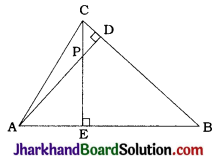1. In ΔAEP and ΔCDP,
∠AEP = ∠CDP (Right angles)
∠EPA = ∠DPC (Vertically opposite angles)
∴ By AA criterion, ΔAEP ~ ΔCDP.

2. In ΔABD and ΔCBE,
∠ABD = ∠CBE (Same angle)
∴ By AA criterion, ΔABD ~ ΔCBE.

∠EAP = ∠DAB (Same angle)
∴ By AA criterion, ΔAEP ~ ΔADB.

4. In ΔPDC and ΔBEC,
∠PDC = ∠BEC (Right angles)
∠PCD = ∠BCE (Same angle)
∴ By AA criterion, ΔPDC ~ ΔBEC.Question 8.
E is a point on the side AD produced of a parallelogram ABCD and BE intersects CD at F. Show that ΔABE ~ ΔCFB.
Given: E is a point on the side AD produced of parallelogram ABCD and BE intersects CD at F
To prove : ΔABE ~ ΔCFB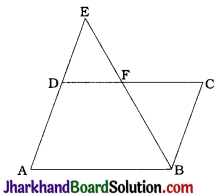Proof: In parallelogram ABCD,
∠A = ∠C (Opposite angles)
∴ ∠BAE = ∠FCB ………..(1)
E lies on AD extended in parallelogram ABCD.
∴ AE || BC
∴ ∠AEB = ∠CBE (Alternate angles)
∴ ∠AEB = ∠CBF …………..(2)
Now, in ΔABE and ΔCFB,
∠BAE = ∠FCB [By (1)]
∠AEB = ∠CBF [By (2)]
∴ By AA criterion, ΔABE ~ ΔCFB.

Question 9.
In the given figure, ABC and AMP are two right triangles, right-angled at B and M respectively. Prove that :
1. ΔABC ~ ΔAMP
2. $$\frac{CA}{PA}=\frac{BC}{MP}$$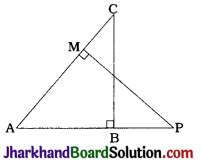In ΔABC and ΔAMP
∠ABC = ∠AMP (Right angles)
∠BAC = ∠MAP (Same angle)
∴ By AA criterion, ΔABC ~ ΔAMP [Result (1)]
Since ΔABC ~ ΔAMP, $$\frac{CA}{PA}=\frac{BC}{MP}$$ [Result (2)]

Question 10.
CD and GH are respectively the bisectors of ∠ACB and ∠EGF such that D and H lie on sides AB and FE of ΔABC and ΔEFG respectively. If ΔABC – ΔFEG, show that:
1. $$\frac{CD}{GH}=\frac{AC}{FG}$$
2. ΔDCB ~ ΔHGE
3. ΔDCA ~ ΔHGF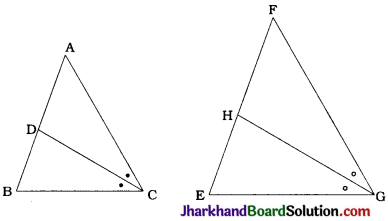ΔABC ~ ΔFEG
∴ ∠A = ∠F, ∠B = ∠E and ∠ACB = ∠FGE …………….(1)
CD is the bisector of ∠ACB and GH is the bisector of ∠FGE.
∴ ∠ACD = ∠BCD = $$\frac{1}{2}$$∠ACB ……(2)
and ∠FGH = ∠EGH = $$\frac{1}{2}$$∠FGE ………..(3)
So, from (1), (2) and (3).
∠ACD = ∠FGH and ∠BCD = ∠EGH ……(4)
Now, in ΔDCB and ΔHGE,
∠B = ∠E [By (1)]
∠BCD = ∠EGH [By (4)]
Hence, by AA criterion,
ΔDCB ~ ΔHGE [Result (2)]
Again, in ΔDCA and ΔHGF
∠A = ∠F [By (1)]
∠ACD = ∠FGH [By (4)]
Hence, by AA criterion,
ΔDCA – ΔHGF [Result (3)]
Now, ΔDCA – ΔHGF
∴ $$\frac{CD}{GH}=\frac{AC}{FG}$$ [Result (1)]Question 11.
In the given figure, E is a point on side CB produced of an isosceles triangle ABC with AB = AC. If AD ⊥ BC and EF ⊥ AC, prove that ΔABD ~ ΔECF.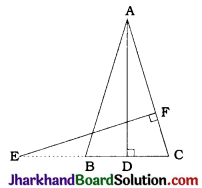In ΔABC, AB = AC
∴ ∠ABC = ∠ACB
∴ ∠ABD = ∠ECF (∵ E lies on CB extended and F lies on AC.)
EF ⊥ AC
∴ ∠EFC = 90°
Now, in ΔABD and ΔECF
∠ABD = ∠ECF
∠ADB = ∠EFC (Both right angles)
∴ By AA criterion, ΔABD ~ ΔECF

Question 12.
Sides AB and BC and median AD of a triangle ABC are respectively proportional to sides PQ and QR and median PM of ΔPQR (see the given figure). Show that ΔABC ~ ΔPQR.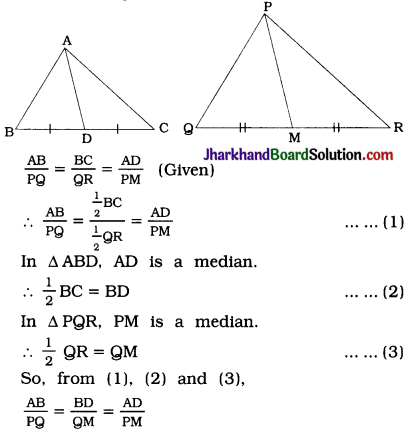Hence, by SSS criterion, ΔABD ~ ΔPQM.
∴ ∠ABD = ∠PQM
∴ ∠ABC = ∠PQR
Now, in ΔABC and ΔPQR,
$$\frac{AB}{PQ}=\frac{BC}{QR}$$ and ∠ABC = ∠PQR
Hence, by SAS criterion, ΔABC ~ ΔPQR.

Question 13.
D is a point on the side BC of a triangle ABC such that ∠ADC = ∠BAC. Show that CA² = CB. CD.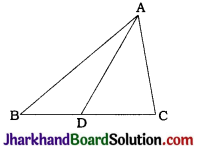In ΔCDA and ΔCAB.
∠ACD = ∠BCA (Same angle)
∴ By AA criterion, ΔCDA ~ ΔCAB
∴ $$\frac{CD}{CA}=\frac{CA}{CB}$$
∴ CB . CD = CA . CA
∴ CA² = CB.CDQuestion 14.
Sides AB and AC and median AD of a triangle ABC are respectively proportional to sides Pg and PR and median PM of another triangle PQR. Show that ΔABC ~ ΔPQR.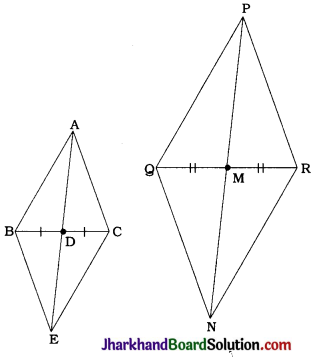In ΔABC, AD is a median.
∴ BD = DC
Take point E on AD extended such that AD = DE and draw BE and CE.
In quadrilateral ABEC, diagonals AE and BC bisect each other.
∴ ABEC is a parallelogram.
∴ BE = AC (Opposite sides) ……(1)
Similarly, in ΔPQR, PM is a median.
∴ QM = MR
Take point N on PM extended such that PM MN and draw QN and RN.
∴ PN = 2PM
In quadrilateral PQNR, diagonals PN and QR bisect each other.
∴ PQNR is a parallelogram.
∴ QN = PR (Opposite sides) …………..(2)
Now,
∴$$\frac{\mathrm{AB}}{\mathrm{PQ}}=\frac{\mathrm{AC}}{\mathrm{PR}}=\frac{\mathrm{AD}}{\mathrm{PM}}$$ (Given)
∴ $$\frac{\mathrm{AB}}{\mathrm{PQ}}=\frac{\mathrm{BE}}{\mathrm{QN}}=\frac{2 \mathrm{AD}}{2 \mathrm{PM}}$$ [By (1) and (2)]
∴$$\frac{AB}{PQ}=\frac{\mathrm{BE}}{\mathrm{QN}}=\frac{\mathrm{AE}}{\mathrm{PN}}$$
∴ By SSS criterion ΔABE ~ ΔPQN.
∴ ∠BAE = ∠QPN
In the same manner, it can be proved that ΔACE ~ ΔPRN
∴ ∠CAE = ∠RPN
∴ ∠BAC = ∠QPR
Now, in ΔABC and ΔPQR,
$$\frac{AB}{PQ}=\frac{AC}{PR}$$ and ∠BAC = ∠QPR
Hence, by SAS criterion, ΔABC ~ ΔPQR.

Question 15.
A vertical pole of length 6 m casts a shadow 4 m long on the ground and at the same. time a tower casts a shadow 28 m long. Find the height of the tower.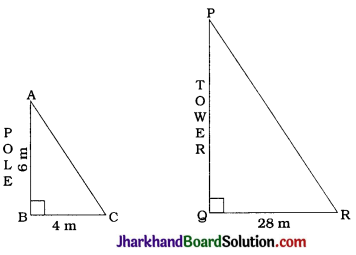Here, AB is the vertical pole and AC is its shadow while PQ is the tower and QR is its shadow.
As both the shadows are measured at the same time, ∠C and ∠R both represent the elevation of the sun.
∴ ∠C = ∠R
In ΔABC and ΔPQR,
∠C = ∠R
∠B = ∠Q (Right angles)
∴ By AA criterion, ΔABC ~ ΔPQR.
∴ $$\frac{AB}{PQ}=\frac{BC}{QR}$$
∴ $$\frac{6}{PQ}=\frac{4}{28}$$
∴ PQ = $$\frac{6 \times 28}{4}$$
∴ PQ = 42 m
Thus, the height of the tower is 42 m.Question 16.
If AD and PM are medians of triangles ABC and PQR respectively; where ΔABC ~ ΔPQR prove that $$\frac{AB}{PQ}=\frac{AD}{PM}$$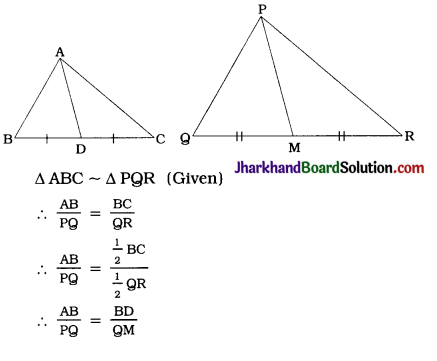$$\frac{AB}{PQ}=\frac{BD}{QM}$$ and ∠ABD = ∠PQM
∴ $$\frac{AB}{PQ}=\frac{AD}{PM}$$